Courses

# Test: Line & Bar Charts - 2

## 15 Questions MCQ Test Topic-wise Past Year Questions for CAT | Test: Line & Bar Charts - 2

Description
This mock test of Test: Line & Bar Charts - 2 for CAT helps you for every CAT entrance exam. This contains 15 Multiple Choice Questions for CAT Test: Line & Bar Charts - 2 (mcq) to study with solutions a complete question bank. The solved questions answers in this Test: Line & Bar Charts - 2 quiz give you a good mix of easy questions and tough questions. CAT students definitely take this Test: Line & Bar Charts - 2 exercise for a better result in the exam. You can find other Test: Line & Bar Charts - 2 extra questions, long questions & short questions for CAT on EduRev as well by searching above.
QUESTION: 1

### Directions for Question: Answer the questions on the basis of the information given below. There are ten real numbers A, B, C, D, E, F, G, H, I. Differences between any two of them are given in the diagram below. (2014)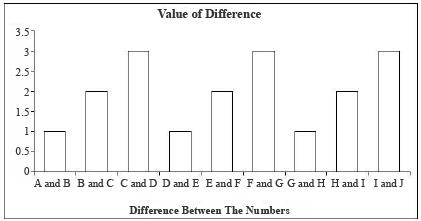Q. If the value of A is known then how many distinct values are possible for J?

Solution:

The table below gives all the possible values of B, C, D, E, F, G, H, l and J if the value of A is assumed to be 'x'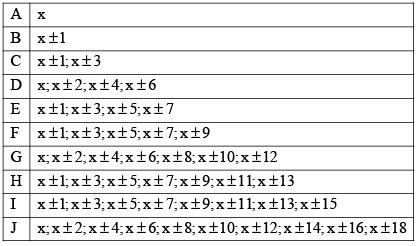Clearly, if the value of A is known then 19 distinct values are possible for J.

QUESTION: 2

### Directions for Question: Answer the questions on the basis of the information given below. There are ten real numbers A, B, C, D, E, F, G, H, I. Differences between any two of them are given in the diagram below. (2014)Q. If all the 10 numbers from A to J are positive integers then at least how many of them are even?

Solution:

The table below gives all the possible values of B, C, D, E, F, G, H, l and J if the value of A is assumed to be 'x'If, 'x' is even then A D. G and J are even. If 'x' is odd then B, C E F, H and I are even.
So, four numbers A, D, G and J are even.

QUESTION: 3

### Directions for Question: Answer the questions on the basis of the information given below. There are ten real numbers A, B, C, D, E, F, G, H, I. Differences between any two of them are given in the diagram below. (2014)Q. If all the 10 numbers from A to J are positive integers and A is equal to 1 then at a time at most how many of them can be perfect squares?

Solution:

The table below gives all the possible values of B, C, D, E, F, G, H, l and J if the value of A is assumed to be 'x'If x = 1, then a possibility is that B = 2, C = 4, D = 1, E = 2, F = 4, G = 1, H = 2,  I = 4 and J = 1 in which case 7 values are perfect squares.
This is the maximum number of perfect squares which can occur at the same time i.e. in a single case.

QUESTION: 4

Directions for Question: Answer the questions on the basis of the information given below.

The average annual salary figures of five leading B-schools have been shown below.
Average Annual Salary (₹ in lakh)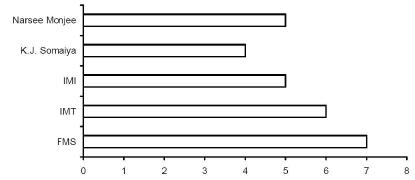The percentage of students getting PPOs (Pre-Placement offers) and their average annual salary in lakhs is shown below: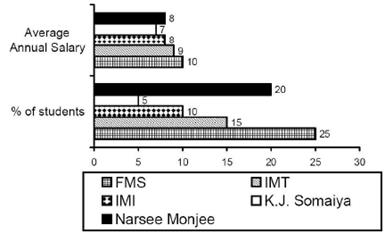The number of students, the number of companies visiting the campus and total offers made (including PPO’s) have been shown below for these five leading B-schools.

(2014)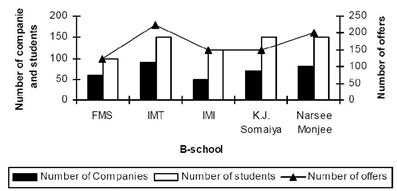Q. Which school has the highest total number of offers per student?

Solution: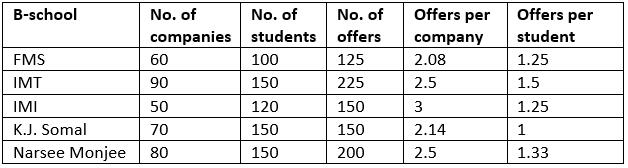IMT has the highest number of offers per student.

QUESTION: 5

Directions for Question: Answer the questions on the basis of the information given below.

The average annual salary figures of five leading B-schools have been shown below.
Average Annual Salary (₹ in lakh)The percentage of students getting PPOs (Pre-Placement offers) and their average annual salary in lakhs is shown below:The number of students, the number of companies visiting the campus and total offers made (including PPO’s) have been shown below for these five leading B-schools.

(2014)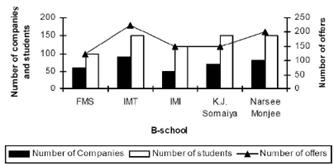Q. The ratio of number of offers to the number of companies visiting the campus is highest for:

Solution:According to above table, IMT has the highest ratio of offers per company.

QUESTION: 6

Directions for Question: Answer the questions on the basis of the information given below.

The average annual salary figures of five leading B-schools have been shown below.
Average Annual Salary (₹ in lakh)The percentage of students getting PPOs (Pre-Placement offers) and their average annual salary in lakhs is shown below:The number of students, the number of companies visiting the campus and total offers made (including PPO’s) have been shown below for these five leading B-schools.

(2014)Q. At FMS, what is the average salary of students, who did not get a PPO?

Solution:Total salary for FMS = 100 × 7 = ₹ 700 lakh
Total salary of PPO holders = (25% of 100) × 10
= 250 lakh
∵ Total salary of people not getting PPOs = 700 – 250
= 450
∵ Average annual salary of people not getting PPOs
= 450 / 75 = 6 lakhs.

QUESTION: 7

Directions for Question: Answer the questions on the basis of the information given below.

The graphs given below show the revenues and profits of four IT education companies.
Profitability = (Profit/Revenue)

(2014)

Total cost = Revenue – Profit Revenues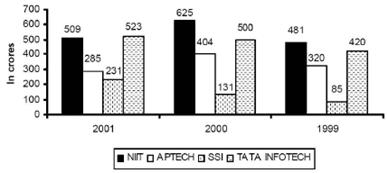Profits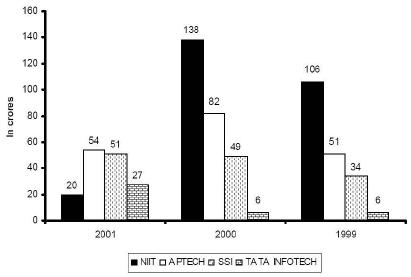Q. In 1999, how many companies have a profitability less than the average of the profitabilities of the four companies?

Solution:

∴ Profitability = Profit / Revenue
Profitability of NIIT in 1999 = 106 / 481 = 0.2204
Profitability of Aptech in 1999 = 51 / 320 = 0.1594
Profitability of  SSI in 1999 = 34 / 85 = 0.4
Profitability of  Tata Infotech in 1999 = 6 / 420 = 0.0143
∴ Average of the profitabilities of the four companies in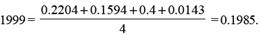∴ Only two companies Tata Infotech and Aptech have a profitability less than the average of the profitabilities in 1999.

QUESTION: 8

Directions for Question: Answer the questions on the basis of the information given below.

The graphs given below show the revenues and profits of four IT education companies.
Profitability = (Profit/Revenue)

(2014)

Total cost = Revenue – Profit RevenuesProfitsQ. In 2002, if the cost in each company increased by 10% over 2001 and the revenue for each company decreased by 10% over 2001, what is the approximate profitability of all the companies taken together in 2002?

Solution:

Total revenues of four companies in 2001 = 509 + 285 + 231 + 523
= 1548 crore
Total profit of four companies in 2001 = 20 + 54 + 51 + 27 = 152 crore
∵ Total cost = Revenue – Profit
∴ Total cost = (1548 – 152) = 1396 crore In 2002, total revenue ≈  (1548 – 155) crore
After decrease by 10% = 1393 crore.
Total cost = (1396 + 140) = 1536 crore
After increasing by 10%
Hence, net profit = (1393 – 1536) = – 143 crore
∴ Profitability = -143 / 1393 = -10.25%

QUESTION: 9

Directions for Question: Answer the questions on the basis of the information given below.

The graphs given below show the revenues and profits of four IT education companies.
Profitability = (Profit/Revenue)

(2014)

Total cost = Revenue – Profit RevenuesProfitsQ. Arrange the companies in increasing order of their profitability in 2001.

Solution:

The profitability of NIIT, Aptech, SSI and Tata lnfotech in 2001 is 20 / 509 = 0.039, 54 / 285 = 0.189, 51 / 231 = 0.220 and 27 / 523 = 0.051 respectively.
Thus, the increasing order is NIIT, Tata Infotech, Aptech and SSI.

QUESTION: 10

Directions for Question: Answer the questions on the basis of the information given below.

The graphs given below show the revenues and profits of four IT education companies.
Profitability = (Profit/Revenue)

(2014)

Total cost = Revenue – Profit RevenuesProfitsQ. Which company has the highest profitability in 2000?

Solution:

The profitability of NIIT in 2000 = 138 / 625 = 0.2208
The profitability of Aptech in 2000 = 82 / 404 = 0.2029
The profitability of SSI in 2000 = 49 / 131 = 0.3740
The profitability of Tata Infotech in 2000 = 6 / 500 = 0.012
So SSI company has the highest profitability in 2000.

QUESTION: 11

Directions for Question: Answer the questions on the basis of the information given below.

The bar graphs given below show the gender-wise deaths (in ‘000) due to NCDs (Non Communicable Diseases) in five countries for the years 2008 and 2009.

(2013)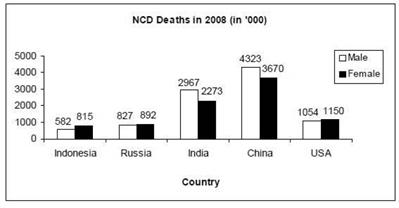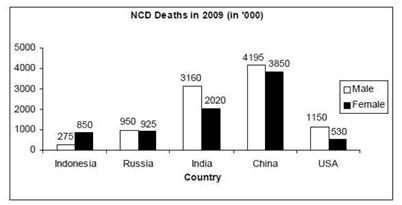Q. In how many countries was the number of deaths due to NCDs in 2009 less than that in 2008?

Solution:

Gender wise deaths due to NCDs in five countries for 2008 and 2009.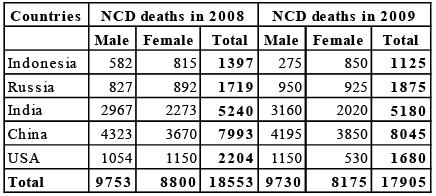Indonesia, India and USA, were the number of deaths due to NCDs in 2009 was less than that in 2008.

QUESTION: 12

Directions for Question: Answer the questions on the basis of the information given below.

The bar graphs given below show the gender-wise deaths (in ‘000) due to NCDs (Non Communicable Diseases) in five countries for the years 2008 and 2009.

(2013)Q. By what percent was the total number of female deaths due to NCDs in the five countries put together in 2009 more/less than that in 2008?

Solution:

Gender wise deaths due to NCDs in five countries for 2008 and 2009.Total number of female death due to NCDs in five countries in 2008 = 8800
Total number of Female death due to NCDs in five countries in 2009 = 8175
∴ Required percentage = 8800 - 8175 / 8800 x 100
= 7.10% (decrease)

QUESTION: 13

Directions for Question : Answer the questions on the basis of the information given below.

The bar graphs given below show the gender-wise deaths (in ‘000) due to NCDs (Non Communicable Diseases) in five countries for the years 2008 and 2009.

(2013)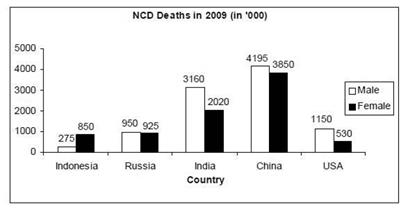Q. What was the absolute difference between the total number of male deaths due to NCDs in the five countries put together in 2008 and 2009?

Solution:

Gender wise deaths due to NCDs in five countries for 2008 and 2009.Total number of male death due to NCDs in five countries in 2008 = 9753
Total number of male death due to NCDs in five countries in 2009 = 9730
∴ Required difference = (9753 – 9730) × 1000 = 23000.

QUESTION: 14

Directions for Question: The following network gives details about the various activities carried out in a bottling firm for their latest project and the time required for each activity. The average cost incurred in each activity is 5 times the square of the duration of the activity. If the organisation wants to reduce the duration of any particular activity, in addition to the average cost, it will have to incur an amount equal to 15 times the cube of the new duration of the activity.

(2011)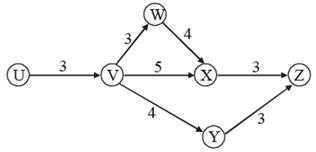Q. The completion of one cycle of the network results in one bottle ready to be sold in the market. The project involves a total of 800 bottles. What is the average cost of the entire project?

Solution:

Average cost of each bottle = 5[32 + 32 + 4+ 52 + 32 + 4+ 32 ]
= 5[9 + 9 + 16 + 25 + 9 + 16 + 9] = 5 x 93 = 465
Average cost of the project = 465 x 800 = 372000 .

QUESTION: 15

Directions for Question: The following network gives details about the various activities carried out in a bottling firm for their latest project and the time required for each activity. The average cost incurred in each activity is 5 times the square of the duration of the activity. If the organisation wants to reduce the duration of any particular activity, in addition to the average cost, it will have to incur an amount equal to 15 times the cube of the new duration of the activity.

(2011)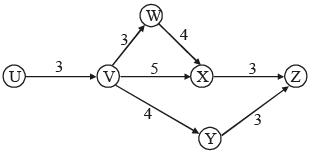Q. If profit is defined as the difference between the selling price and the average cost, and each bottle is sold for ₹ 510, what is the approximate percent profit earned by the firm?

Solution:

Profit % = 510 - 465 / 465 x 100 = 45 / 465 x 100
= 9.67 ≈ 10%